Home | | Maths 12th Std | Choose the correct Answer

## Chapter: 12th Mathematics : UNIT 5 : Two Dimensional Analytical Geometry II

Choose the correct or the most suitable answer from the given four alternatives, Multiple choice questions with Answers, Solution - Maths Book back 1 mark questions and answers with solution for Exercise Problems

Choose the correct or the most suitable answer from the given four alternatives :

1. The equation of the circle passing through (1, 5) and (4,1) and touching -axis is xy− 5− 6 + 9 + λ (4+ 3−19) = 0 where λ is equal to

(1) 0, - 40/9

(2) 0

(3) 40/9

(4) -40/92. The eccentricity of the hyperbola whose latus rectum is 8 and conjugate axis is equal to half the distance between the foci is

(1) 4/3

(2) 4/√3

(3) 2√3

(4) 3/23. The circle xy= 4+ 8 + 5 intersects the line 3− 4 at two distinct points if

(1) 15 < m < 65

(2) 35 < m < 85

(3) -85 < m < -35

(4) -35 < m < 154. The length of the diameter of the circle which touches the -axis at the point (1,0) and passes through the point (2, 3) .

(1) 6/5

(2) 5/3

(3) 10/3

(4) 3/55. The radius of the circle 3xby+ 4bx − 6by b= 0 is

(1) 1

(2) 3

(3) √10

(3) √11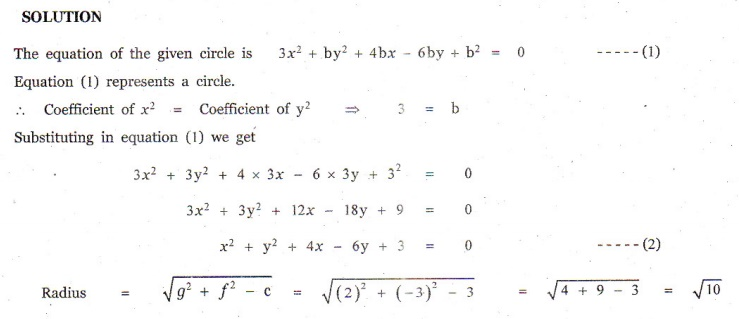6. The centre of the circle inscribed in a square formed by the lines x− 8−12 = 0 and  y−14 + 45 = 0 is

(1) (4, 7)

(2) (7, 4)

(3) (9, 4)

(4) (4, 9)7. The equation of the normal to the circle xy− 2− 2 +1 = 0 which is parallel to the line 2+ 4 = 3 is

(1) x + 2 y = 3

(2) x + 2 y + 3 = 0

(3) 2 x + 4 y + 3 = 0

(4) x - 2 y + 3 = 08. If P(xy) be any point on 16x+ 25 y= 400 with foci F(3, 0) and F(−3, 0) then PFPF2 is

(1) 8

(2) 6

(3) 10

(4) 129. The radius of the circle passing through the point (6, 2) two of whose diameter are = 6 and + 2 = 4 is

(1) 10

(2) 2√5

(3) 6

(4) 410. The area of quadrilateral formed with foci of the hyperbolas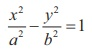andis

(1) 4(a2 + b2 )

(2) 2(a2 + b2 )

(3) a2 + b2

(4) 1/2 (a2 + b2 )11. If the normals of the parabola y= 4drawn at the end points of its latus rectum are tangents to the circle (− 3)+ ( + 2), then the value of is

(1) 2

(2) 3

(3) 1

(4) 412. If is a normal to the parabola y= 12, then the value of is

(1) 3

(2) -1

(3) 1

(4) 913. The ellipse Eis inscribed in a rectangle whose sides are parallel to the coordinate axes. Another ellipse Epassing through the point (0, 4) circumscribes the rectangle . The eccentricity of the ellipse is

(1) √2/2

(2) √3/2

(3) 1/2

(4) 3/414. Tangents are drawn to the hyperbolaparallel to the straight line 2− = 1. One ofthe points of contact of tangents on the hyperbola isAns: (3)15. The equation of the circle passing through the foci of the ellipsehaving centre at (0,3) is

(1) x2 y2 - 6 - 7 = 0

(2) x2 y2 - 6 + 7 = 0

(3) x2 + y2 - 6 y - 5 = 0

(4) x2 + y2 - 6 y + 5 = 0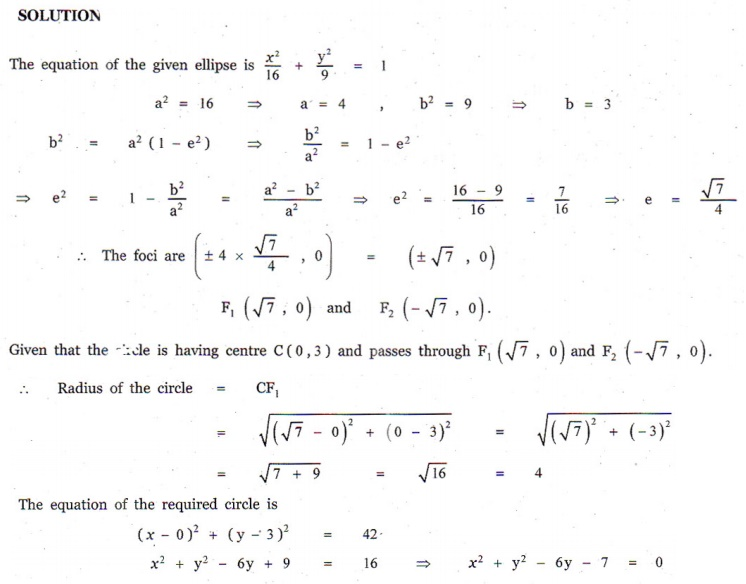16. Let be the circle with centre at (1,1) and radius = 1 . If is the circle centered at (0, y) passing through the origin and touching the circle Cexternally, then the radius of is equal to

(1)  √3/√2

(2) √3/2

(3) 1/2

(4) 1/417. Consider an ellipse whose centre is of the origin and its major axis is along x-axis. If its eccentrcity is 3/5 and the distance between its foci is 6, then the area of the quadrilateral inscribed in the ellipse with diagonals as major and minor axis of the ellipse is

(1) 8

(2) 32

(3) 80

(4) 4018. Area of the greatest rectangle inscribed in the ellipse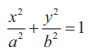is

(1) 2ab

(2) ab

(3) √ab

(4) a/b19. An ellipse has OB as semi minor axes, and ′ its foci and the angle FBF ′ is a right angle. Then the eccentricity of the ellipse is

(1) 1/√2

(2) 1/2

(3) 1/4

(4) 1/√320. The eccentricity of the ellipse (x - 3)2 + ( y - 4)2 = y2/9 is

(1)  √3 / 2

(2) 1 / 3

(3) 1 / 3√2

(4) 1 / √3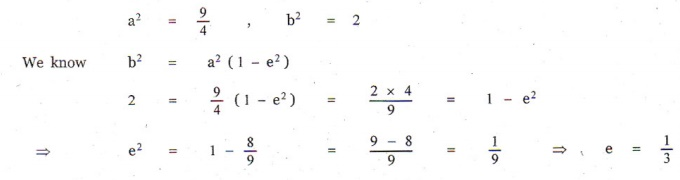21. If the two tangents drawn from a point to the parabola y= 4are at right angles then the locus of is

(1) 2x +1 = 0

(2) x = -1

(3) 2x -1 = 0

(4) x = 122. The circle passing through (1, −2) and touching the axis of at (3, 0) passing through the point

(1) (-5, 2)

(2) (2, -5)

(3) (5, -2)

(4) (-2, 5)23. The locus of a point whose distance from (−2, 0) is 2/3 times its distance from the line x=-9/2 is

(1) a parabola

(2) a hyperbola

(3) an ellipse

(4) a circle24. The values of for which the line y = mx + 2√5  touches the hyperbola 16x− 9 y= 144 are the roots of x− (b)− 4 = 0 , then the value of (b) is

(1) 2

(2) 4

(3) 0

(4) -225. If the coordinates at one end of a diameter of the circle xy− 8− 4 = 0 are (11, 2) , the coordinates of the other end are

(1) (-5, 2)

(2) (2, -5)

(3) (5, -2)

(4) (-2, 5)Choose the correct or the most suitable answer from the given four alternatives :

1. The equation of the circle passing through (1, 5) and (4,1) and touching -axis is xy− 5− 6 + 9 + λ (4+ 3−19) = 0 where λ is equal to

(1) 0, - 40/9

(2) 0

(3) 40/9

(4) -40/9

2. The eccentricity of the hyperbola whose latus rectum is 8 and conjugate axis is equal to half the distance between the foci is

(1) 4/3

(2) 4/√3

(3) 2√3

(4) 3/2

3. The circle xy= 4+ 8 + 5 intersects the line 3− 4 at two distinct points if

(1) 15 < m < 65

(2) 35 < m < 85

(3) -85 < m < -35

(4) -35 < m < 15

4. The length of the diameter of the circle which touches the -axis at the point (1,0) and passes through the point (2, 3) .

(1) 6/5

(2) 5/3

(3) 10/3

(4) 3/5

5. The radius of the circle 3xby+ 4bx − 6by b= 0 is

(1) 1

(2) 3

(3) √10

(3) √11

6. The centre of the circle inscribed in a square formed by the lines x− 8−12 = 0 and  y−14 + 45 = 0 is

(1) (4, 7)

(2) (7, 4)

(3) (9, 4)

(4) (4, 9)

7. The equation of the normal to the circle xy− 2− 2 +1 = 0 which is parallel to the line 2+ 4 = 3 is

(1) x + 2 y = 3

(2) x + 2 y + 3 = 0

(3) 2 x + 4 y + 3 = 0

(4) x - 2 y + 3 = 0

8. If P(xy) be any point on 16x+ 25 y= 400 with foci F(3, 0) and F(−3, 0) then PFPF2 is

(1) 8

(2) 6

(3) 10

(4) 12

9. The radius of the circle passing through the point (6, 2) two of whose diameter are = 6 and + 2 = 4 is

(1) 10

(2) 2√5

(3) 6

(4) 4

10. The area of quadrilateral formed with foci of the hyperbolasandis

(1) 4(a2 + b2 )

(2) 2(a2 + b2 )

(3) a2 + b2

(4) 1/2 (a2 + b2 )

11. If the normals of the parabola y= 4drawn at the end points of its latus rectum are tangents to the circle (− 3)+ ( + 2), then the value of is

(1) 2

(2) 3

(3) 1

(4) 4

12. If is a normal to the parabola y= 12, then the value of is

(1) 3

(2) -1

(3) 1

(4) 9

13. The ellipse Eis inscribed in a rectangle whose sides are parallel to the coordinate axes. Another ellipse Epassing through the point (0, 4) circumscribes the rectangle . The eccentricity of the ellipse is

(1) √2/2

(2) √3/2

(3) 1/2

(4) 3/4

14. Tangents are drawn to the hyperbolaparallel to the straight line 2− = 1. One ofthe points of contact of tangents on the hyperbola isAns: (3)

15. The equation of the circle passing through the foci of the ellipsehaving centre at (0,3) is

(1) x2 y2 - 6 - 7 = 0

(2) x2 y2 - 6 + 7 = 0

(3) x2 + y2 - 6 y - 5 = 0

(4) x2 + y2 - 6 y + 5 = 0

16. Let be the circle with centre at (1,1) and radius = 1 . If is the circle centered at (0, y) passing through the origin and touching the circle Cexternally, then the radius of is equal to

(1)  √3/√2

(2) √3/2

(3) 1/2

(4) 1/4

17. Consider an ellipse whose centre is of the origin and its major axis is along x-axis. If its eccentrcity is 3/5 and the distance between its foci is 6, then the area of the quadrilateral inscribed in the ellipse with diagonals as major and minor axis of the ellipse is

(1) 8

(2) 32

(3) 80

(4) 40

18. Area of the greatest rectangle inscribed in the ellipseis

(1) 2ab

(2) ab

(3) √ab

(4) a/b

19. An ellipse has OB as semi minor axes, and ′ its foci and the angle FBF ′ is a right angle. Then the eccentricity of the ellipse is

(1) 1/√2

(2) 1/2

(3) 1/4

(4) 1/√3

20. The eccentricity of the ellipse (x - 3)2 + ( y - 4)2 = y2/9 is

(1)  √3 / 2

(2) 1 / 3

(3) 1 / 3√2

(4) 1 / √3

21. If the two tangents drawn from a point to the parabola y= 4are at right angles then the locus of is

(1) 2x +1 = 0

(2) x = -1

(3) 2x -1 = 0

(4) x = 1

22. The circle passing through (1, −2) and touching the axis of at (3, 0) passing through the point

(1) (-5, 2)

(2) (2, -5)

(3) (5, -2)

(4) (-2, 5)

23. The locus of a point whose distance from (−2, 0) is 2/3 times its distance from the line x=-9/2 is

(1) a parabola

(2) a hyperbola

(3) an ellipse

(4) a circle

24. The values of for which the line y = mx + 2√5  touches the hyperbola 16x− 9 y= 144 are the roots of x− (b)− 4 = 0 , then the value of (b) is

(1) 2

(2) 4

(3) 0

(4) -2

25. If the coordinates at one end of a diameter of the circle xy− 8− 4 = 0 are (11, 2) , the coordinates of the other end are

(1) (-5, 2)

(2) (2, -5)

(3) (5, -2)

(4) (-2, 5)Tags : Two Dimensional Analytical Geometry II , 12th Mathematics : UNIT 5 : Two Dimensional Analytical Geometry II
Study Material, Lecturing Notes, Assignment, Reference, Wiki description explanation, brief detail
12th Mathematics : UNIT 5 : Two Dimensional Analytical Geometry II : Choose the correct Answer | Two Dimensional Analytical Geometry II